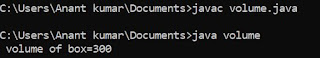## Wednesday, 27 June 2018

### C program to find sum of array of n element

/* program to sum of array */
# include <stdio.h>
void main ()
{
int a , sum=0 , n ;
clrscr () ;
printf ("\n How many no you want to add:") ;
scanf ("%d", &n) ;
if ( n > 20 )
{
printf ("\n Invalid number:") ;
getch () ;
exit () ;
}
for ( i=0 ; i<n ; i++ )
{
printf ("\n Enter a number:") ;
scanf ("%d", &a[n]) ;
}
for ( i=0 ; i<n ; i++ )
sum = sum + a[i] ;
printf ("\n sum =%d", sum) ;
getch () ;
}

PROCESS :

Here we have to input number of element from the user and then we have to write stubs for checking the number of element . If we use studs as number of element as 20 then we have to use element as under as 20 otherwise it becomes invalid number .
As we know that array is example of static data structure in which the size of data structure can not be increased or decreased at run time of program